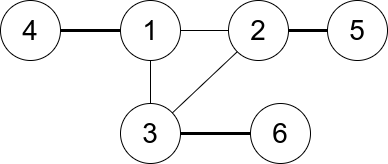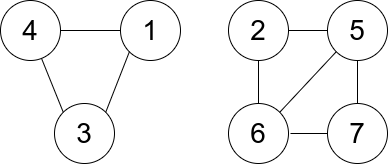##### Welcome to Subscribe On Youtube

Formatted question description: https://leetcode.ca/all/1761.html

# 1761. Minimum Degree of a Connected Trio in a Graph

Hard

## Description

You are given an undirected graph. You are given an integer n which is the number of nodes in the graph and an array edges, where each edges[i] = [u_i, v_i] indicates that there is an undirected edge between u_i and v_i.

A connected trio is a set of three nodes where there is an edge between every pair of them.

The degree of a connected trio is the number of edges where one endpoint is in the trio, and the other is not.

Return the minimum degree of a connected trio in the graph, or -1 if the graph has no connected trios.

Example 1:Input: n = 6, edges = [[1,2],[1,3],[3,2],[4,1],[5,2],[3,6]]

Output: 3

Explanation: There is exactly one trio, which is [1,2,3]. The edges that form its degree are bolded in the figure above.

Example 2:Input: n = 7, edges = [[1,3],[4,1],[4,3],[2,5],[5,6],[6,7],[7,5],[2,6]]

Output: 0

Explanation: There are exactly three trios:

1) [1,4,3] with degree 0.

2) [2,5,6] with degree 2.

3) [5,6,7] with degree 2.

Constraints:

• 2 <= n <= 400
• edges[i].length == 2
• 1 <= edges.length <= n * (n-1) / 2
• 1 <= u_i, v_i <= n
• u_i != v_i
• There are no repeated edges.

## Solution

Use a hash map to store each node’s connected nodes. Then loop over all tuples of nodes and determine whether the three nodes form a connected trio. If the three nodes form a connected trio, calculate the sum of the numbers of edges that connect to the three nodes and subtract 6 from the sum (to exclude the edges inside the trio). During the process, maintain the minimum degree. Finally, return the minimum degree.

• class Solution {
public int minTrioDegree(int n, int[][] edges) {
Map<Integer, Set<Integer>> map = new HashMap<Integer, Set<Integer>>();
for (int[] edge : edges) {
int node0 = edge, node1 = edge;
Set<Integer> set0 = map.getOrDefault(node0, new HashSet<Integer>());
Set<Integer> set1 = map.getOrDefault(node1, new HashSet<Integer>());
map.put(node0, set0);
map.put(node1, set1);
}
int minDegree = Integer.MAX_VALUE;
for (int i = 1; i <= n; i++) {
Set<Integer> set = map.getOrDefault(i, new HashSet<Integer>());
if (set.size() < 2)
continue;
List<Integer> list = new ArrayList<Integer>(set);
int size = list.size();
for (int j = 0; j < size; j++) {
int node1 = list.get(j);
Set<Integer> set1 = map.get(node1);
for (int k = j + 1; k < size; k++) {
int node2 = list.get(k);
if (set1.contains(node2)) {
Set<Integer> set2 = map.get(node2);
int count = set.size() + set1.size() + set2.size() - 6;
minDegree = Math.min(minDegree, count);
}
}
}
}
return minDegree == Integer.MAX_VALUE ? -1 : minDegree;
}
}

• Todo

• class Solution:
def minTrioDegree(self, n: int, edges: List[List[int]]) -> int:
g = [[False] * n for _ in range(n)]
deg =  * n
for u, v in edges:
u, v = u - 1, v - 1
g[u][v] = g[v][u] = True
deg[u] += 1
deg[v] += 1
ans = inf
for i in range(n):
for j in range(i + 1, n):
if g[i][j]:
for k in range(j + 1, n):
if g[i][k] and g[j][k]:
ans = min(ans, deg[i] + deg[j] + deg[k] - 6)
return -1 if ans == inf else ans